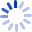# Discrete Mathematics with Applications

Providing an introduction to discrete mathematics, this book presents the major themes of discrete mathematics and the reasoning that underlies mathematical thought. It helps students learn about such concepts as logic circuits and computer addition, algorithm analysis, recursive thinking, computability, automata, cryptography, and combinatorics.

##### Cash for Textbooks